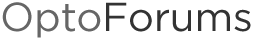# A problem of IEEE754 data conversion

The 4 byte string contains a IEEE754 single precision floating point number. I want to get this floating point number. Is it necessary to first convert one byte to one byte into 16 hexadecimal strings and then convert the sixteen string string to floating point number? Is there a convenient command to get this floating point?

You can try the UnpackString command (It’s one of the HART commands):

`UnpackString(Hex_String, 0, 4, MyFloatVar, 3, 0);`

On older firmware and PAC Control, you can do this:

`Move32Bits((Hex_String << 24) + (Hex_String << 16) + (Hex_String << 8) +(Hex_String), MyFloatVar);`

1 Like

The two methods are easy to handle.
Thank you very much.